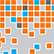# Online Program

Friday, May 31Modern Multivariate Analysis
Fri, May 31, 3:40 PM - 5:15 PM
Regency Ballroom AB

### \$L_2\$-Regularization and Some Path-Following Algorithms (305043)

Renxiong Liu, The Ohio State University
*Yunzhang Zhu, The Ohio State University

Keywords: regularization, path-following algorithm, optimization

We establish an equivalence between \$L_2\$-regularized solution path and the solution to an ordinary differentiable equation. In addition, we show that the solution path is monotone in terms of its \$L_2\$ norm and the empirical loss. Moreover, we show that the limit of the solution path coincides with the minimum \$L_2\$-norm minimizer of the empirical loss provided that it is finite. Importantly, this equivalence result reveals that the solution path can be viewed as the flow of a hybrid of gradient descent and Newton method applying to the empirical loss, which is similar to the trust region method and Levenberg-Marquardt algorithm. This is in contrast to the conventional view that the \$L_2\$ regularization solution path is similar to the gradient flow of the empirical loss. Numerical ODE solvers and optimization-based warm-start strategies are considered to numerically approximate the solution path. In particular, we consider Newton update and gradient descent update in the warm-start strategy, and establish the global approximation error of these two methods. In terms of computational cost, these warm-start strategies are also shown to require roughly the same computational cost required for computing a single solution on the path. Finally, we use \$L_2\$-regularized logistic regression as an example to illustrate the effectiveness of various warm-start strategies.